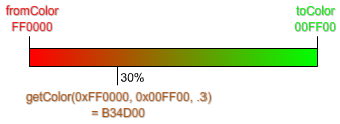## Find the value between two colorsA handy function I wrote that calculates a color value between two colors.

```function getColor(fromColor:uint, toColor:uint, scale:Number):uint {
var red:uint = ((fromColor >> 16) + (toColor >> 16) - (fromColor >> 16) * scale) << 16;
var green:uint = ((fromColor >> 8 & 255) + ((toColor >> 8 & 255) - (fromColor >> 8 & 255)) * scale) << 8;
var blue:uint = (fromColor & 255) + ((toColor & 255) - (fromColor & 255)) * scale;

return red + green + blue;
}```